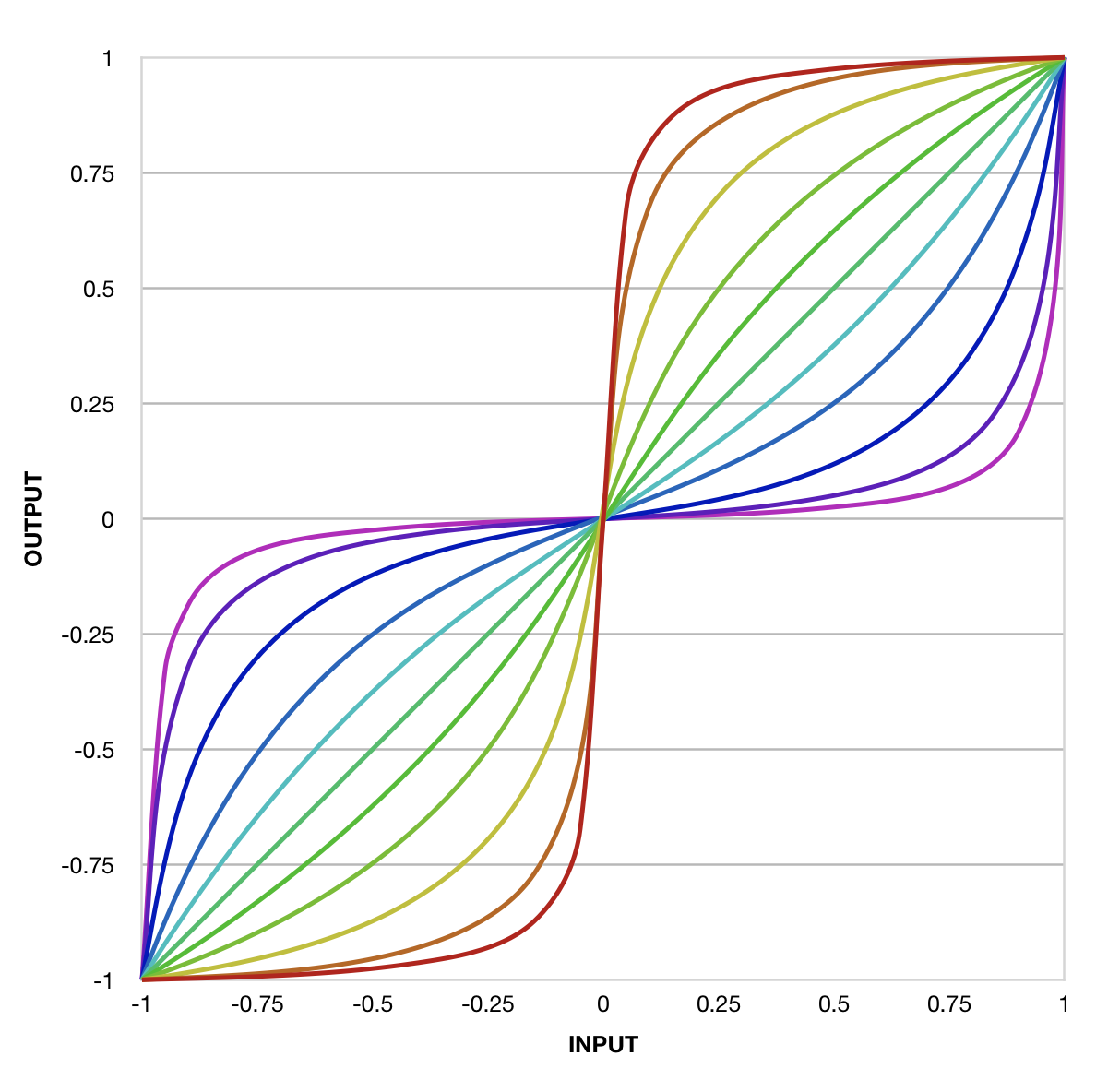# The Function

As I looked for a way to shape envelope stages, I stumbled across Dino Dini’s beautiful normalized tunable sigmoid function:

$$y = \frac{x - kx}{k - 2k|x| + 1}$$

By beautiful, I don’t mean that the function looks nice (though it looks very nice). I mean that it has three features that make it enormously useful for my purposes:

• Normalized: It applies in a particularly useful way to numbers in two particularly useful ranges: [-1,1] and [0,1].
• Tunable: A single parameter (k or curvature) adjusts the curve in particularly useful ways.
• Sigmoid: It produces a particularly useful S-shaped curve. And because of the multiple ways it is normalized, it also produces a particularly useful J-shaped curve.

Here is a graph of the the normalized tunable sigmoid function for inputs from -1 to 1, with each line representing a different curvature, from -0.95 (red) to 0.95 (violet):## Normalized

The function is normalized in two interesting ways:

• Given an input in the range [-1,1], it yields an output in the range [-1,1].
• Given an input in the range [0,1], it yields an output in the range [0,1].

Note also that for every value of k:

• $$sigmoid(-1) = -1$$.
• $$sigmoid(0) = 0$$.
• $$sigmoid(1) = 1$$.

If I can map some linear signal onto one of those “normal” ranges, I can apply the sigmoid function to shape the signal.

This is how the DHE Modules create curved envelope stages and waveshaper transfer functions.

## Tunable

The normalized tunable sigmoid function has a parameter, k, which tunes the curvature of the function. Each line in the graph represents a different value of k.

Positive k (from green to violet in the graph) gives a J shape over the range [0,1] and an inverted S shape over the range [-1,1]. Negative k (from green to red in the graph) gives a shape that is nicely symmetrical to the positive ks in either range.

And when $$k=0$$, the function is linear: $$y=x$$. The output is the same as the input.

By mapping each module’s CURVE knob to this function parameter k, I give users control over the curvature of envelope stages and waveshaper transfer functions.

Note: For S-shaped curves, the DHE Modules negate the tuning parameter k so that turning the CURVE knob clockwise gives an S curve instead of an inverted S curve.

## Sigmoid

Originally I sought a function that could produce useful, symmetrical “logarithmic” curves for envelope stages. My initial attraction to this normalized tunable sigmoid function was that in the range [0,1] it produced exactly the kind of J curve I was looking for.

But playing with this curve got me thinking: What if my modules could produce S shaped envelope stages? That would be a distinguishing feature! And this function made S shaped envelope stages easy.

Then I wondered: Might this function work well as a waveshaper transfer function? And just like that, the idea for Swave was born.

# Curved Envelope Stages

Stage and Booster Stage use the normalised tunable sigmoid function to produce curved envelope stages.

To produce an envelope stage, each module follows the same general procedure:

1. Generate a ramp signal that progresses from 0 to 1 over the duration of the stage
2. Apply a shaping function to shape the ramp signal into a curve
3. Map the curved ramp signal onto the desired output range

For step 2, each module shapes the ramp signal by applying the normalized tunable sigmoid function.

J-Curved Envelope Stages: Given that the ramp signal is already in the range [0,1], shaping the stage into a J curve is easy: simply call the sigmoid function.

S-Curved Envelope Stages: Booster Stage can produce S-shaped stages. To do this, it applies the shaping function in three steps:

1. Map the ramp signal from [0,1] to [-1,1]
2. Apply the sigmoid function
3. Map the shaped signal back to [0,1] before scaling it to the desired output range

# Waveshaping

Swave uses the normalized tunable sigmoid function to shape its input signal.

Note: Swave assumes that its input signal is in the range [-5V,5V], the standard range for audio and bipolar CV signals in VCV Rack.

To shape its input signal, Swave:

1. Maps the signal from [-5V,5V] to the range [0,1] (for J-curved waveshaping) or [-1,1] (for S-curved waveshaping)
2. Applies the sigmoid function to shape the signal
3. Maps the shaped signal back to the range [-5V,5V] for output

# Other Uses

In addition to shaping signals and generating envelope stages, DHE Modules use the sigmoid function to give some knobs a taper, making the knobs more or less senstive at different parts of their rotation:

• Each DURATION knob has a J taper, making it less sensitive near the counterclockwise extreme and more sensitive near the clockwise extreme
• Each CURVE knob has an S taper, making it more sensitive in the center and less sensitive at the extremes